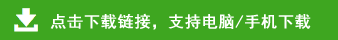# 小学二年级下册数学精选口算题

12-36÷9=        12÷4×3=        56÷8×5=

14÷7+68=        58+35÷7=        9×4+56=
9×8-15=          2÷1+41=        2+23=
29+2×7=        42÷6+35=        64÷8×6=
55+3×9=        75+4×4=        35÷7+57=
1×7+88=        22-20÷5=        68-7×9=
9×7-32=        32÷8-1=            66-6÷2=
81-28÷7=        54÷9+37=        21÷3+75=
4×4÷2=        16÷8-1=        24+12÷2=
3×7-12=        50-9×3=        27÷9-3=
9÷3+63=        83-9×9=        83-25÷5=

27+5×1=        7×5+7=        40÷8-1=
32-8÷2=        40÷8×9=        2×6÷3=
36+8÷1=        8×4+29=        6÷1+35=
9×2÷6=        21÷7+49=        15÷5×8=
67+7÷1=        64+7×4=        42÷7÷2=
58+3×2=        87+14÷2=        31-10÷2=
7+4×9=        63÷9×3=        65-16÷2=
7×8-21=        77+32÷8=        95-6×9=
30÷6×7=        5×7+58=        18÷9-1=
64÷8÷4=        9×6-34=        8×8+9=
95-49÷7=        16+9×6=        4×9÷6=
39+40÷8=        72÷9×8=        30-3×4=
45-5×9=        9×3+27=        7×7+45=
73-7÷1=        8×3+38=        15÷5×9=
39-42÷7=        5×5-17=        79+40÷5=

51-16÷4=        63-24÷3=       42÷6×4=